# Stochastic Calculus: Conditional Expectation

WMDhamnekar
MHB
Homework Statement:
. Suppose we roll two dice, a red and a green one, and let X
be the value on the red die and Y the value on the green die. Let Z = X/Y .
1)Find E[X + 2Y | Z].
2)Let W = E[Z | X]. What are the possible values for W? Give the
distribution of W.
Relevant Equations:
Not applicable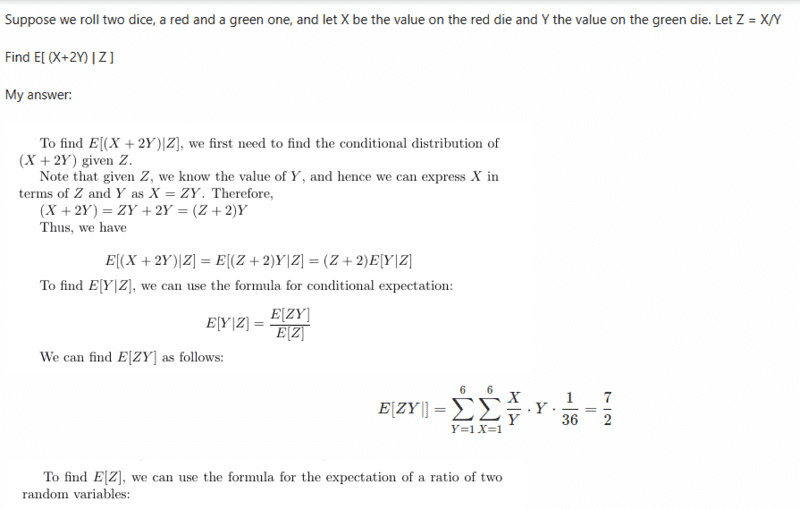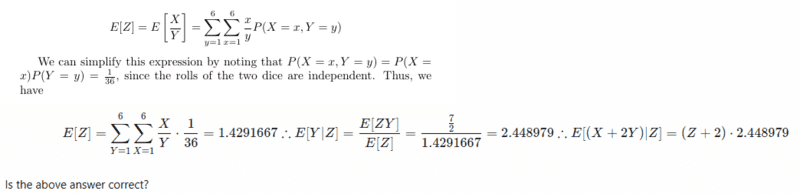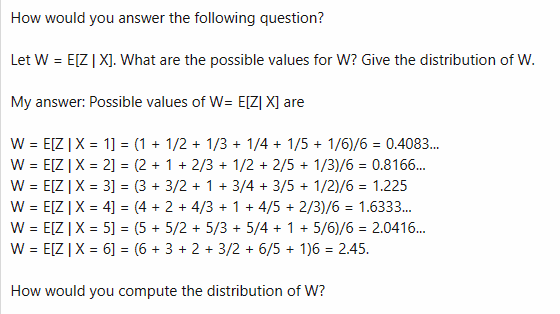Homework Helper
Your answer to 1 is wrong, as you can easily check using some actual values of Z, e.g. for Z = 6, E(X+2Y) = 8.

Your mistake appears to be using global averages for ZY and Z, calculated over all combinations of X and Y. But for a given Z, not all combinations are possible. for example, for Z = 6, 5 or 4, Y can only have the value 1, and X the value of Z. For Z = 1, only combinations with X = Y are possible. Thus E[Y|Z] is not a constant but depends on Z.

•WMDhamnekar
Homework Helper
Gold Member
To specify the distribution for a discrete-valued random variable like W you need to specify the set of unique possible values it can take, and the probability of each value.

You have identified above six possible values of W=E[Z|X], each corresponding to a different result X from the red die. Assuming that die is fair, what is the probability of each of those different values?

One thing remains: you need to check wheter any of the six values of W are the same, ie if two different values of X can give the same value of W. If any do, you need to combine them by adding their probabilities.

•WMDhamnekar
WMDhamnekar
MHB
I prepared two tables. Now how to compute E[X +2Y| Z]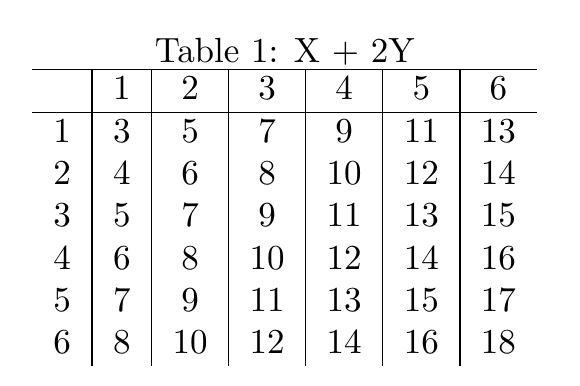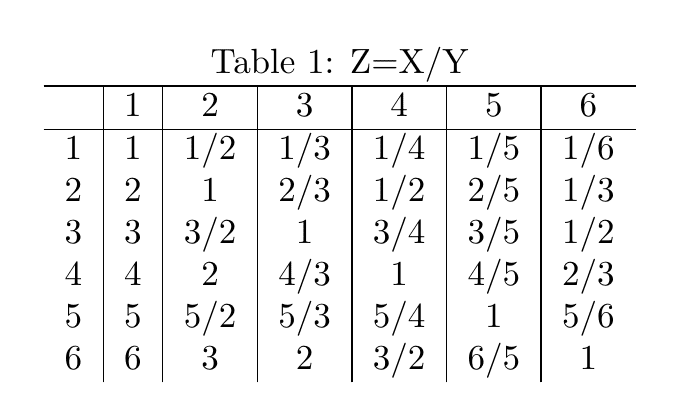Homework Helper
Gold Member
For instance, for Z=1 in the second table, you have 6 equally likely possibilities for (X,Y): (1,1), (2,2), (3,3), (4,4), (5,5), (6,6).
In your first table, that gives: P(X+2Y | Z=1) = P( X+2Y = 3,6,9,12,15,18 | Z=1) = 1/6.

Likewise, for Z=1/2 in the second table, you have 3 equally likely possibilities for (X,Y): (1,2), (2,4), (3,6).
In your first table, that gives: P(X+2Y | Z=1/2) = P( X+2Y = 5,10,15 | Z=1/2) = 1/3.
Etc.
For each value of Z, you get a distribution of X+2Y values that sum to 1.
I don't see a short-cut way to calculate the expected value. I guess you might just have to tediously calculate it.

Gold Member
I'm a bit confused on your notation. Generally, in an expression E(X|Y), Y is an event, and X,Y are events in the same sample space. Maybe you mean E(X| Z=Zo) ?

•FactChecker
WMDhamnekar
MHB
For instance, for Z=1 in the second table, you have 6 equally likely possibilities for (X,Y): (1,1), (2,2), (3,3), (4,4), (5,5), (6,6).
In your first table, that gives: P(X+2Y | Z=1) = P( X+2Y = 3,6,9,12,15,18 | Z=1) = 1/6.

Likewise, for Z=1/2 in the second table, you have 3 equally likely possibilities for (X,Y): (1,2), (2,4), (3,6).
In your first table, that gives: P(X+2Y | Z=1/2) = P( X+2Y = 5,10,15 | Z=1/2) = 1/3.
Etc.
For each value of Z, you get a distribution of X+2Y values that sum to 1.
I don't see a short-cut way to calculate the expected value. I guess you might just have to tediously calculate it.
E[ X + 2Y | Z] = [3 + 6 + 9 + 12 + 15+ 18 =63]* 1/6 = 10.5 + [5 +10+15]*1/12= 2.5 + [7+ 14]*1/18 =1.1667 + [9 + 11 + 13 +12 + 11+ 13+ 6 + 10 +14 + 7 +9 + 11 +13 +17 + 8 +16 =179]*1/36 = 4.9722222 +[4 +8 +12=24]*1/12=2 + [ 8 +16=24]*1/18 = 1.33333 +[5 + 10 = 15]*1/18 =0.8333333 + [7 +14=21]*1/18 = 1.1667 = 24.47223

Homework Helper
Gold Member
I guess that's right (I haven't checked your calculations). But I do have the same question as @WWGD.
Does the notation E(X+2Y | Z) mean the function, f(z), of z, f(z) = E(X+2Y | Z=z)? Or does it mean that you take an expected value over all possible values of Z as you did?

•WWGD
WMDhamnekar
MHB
I guess that's right (I haven't checked your calculations). But I do have the same question as @WWGD.
Does the notation E(X+2Y | Z) mean the function, f(z), of z, f(z) = E(X+2Y | Z=z)? Or does it mean that you take an expected value over all possible values of Z as you did?
Suppose we roll two dice, one is red one and another one is green one. If we let the values on flipping the two dice be X and Y. ( X for the value on red die and Y for the value on green die). Then obviously X and Y values both lies in the same sample space and Z, the function of X and Y will take the value Z = X/Y.